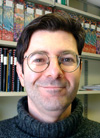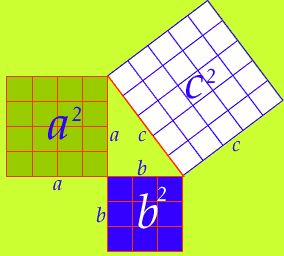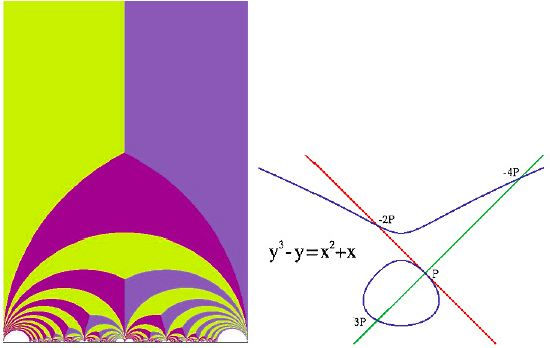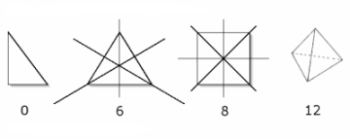# Henri Darmon Pure and Applied Mathematics

One of the world's leading number theorists, working on Hilbert's 12th problem.

"Somewhere out there is a theory that would explain my empirical observations, and this theory has yet to be discovered. Mathematics thrives on such mysteries."

## The Story

Henri Darmon and his girlfriend, Galia, were driving back to Princeton, New Jersey, on a warm Sunday in June after a holiday at Cape Cod. The 20-something couple had been together for two years. They had met in 1991 when Darmon arrived at Princeton University to work as a mathematics instructor and researcher; Galia was studying mathematics as well, finishing her doctorate (PhD).

As mathematicians often do, Henri and Galia whiled away the miles by musing about some of the big puzzles nobody could solve. “So what about Fermat’s last theorem?” asked Galia. “Do you think anyone will ever prove it?” She was referring to a seemingly simple problem that Darmon knew a lot about. His own research on elliptic curves might someday be involved in a mathematical proof. Over the centuries, millions of people had tried to solve French mathematician Pierre de Fermat’s last theorem, which he had scribbled in the margin of a book in 1637, but nobody could do it.

A mathematical theorem is a truth derived from fundamental principles. Fermat’s last theorem relates to another classic phenomenon called the Pythagorean theorem, which says: for the sides of a right triangle, the sum of the squares of the two short sides equals the square of the long side. The figure opposite demonstrates this idea, which was actually discovered by the Babylonians (the ancient civilization that existed in what is now called Iraq) around 2000 b.c. They used it to figure out the relative value of farm fields for tax purposes, but it is attributed to the famous Greek mathematician Pythagoras, who studied it in great detail.Usually the theorem is stated algebraically: a2 + b2 = c2, where c is the hypotenuse (long side) and a and b are the short sides of the triangle. Lots of numbers satisfy this rule starting with 3, 4 and 5 (replace the b with 3, the a with 4 and the c with 5. Then you get 32 = 9, 42 = 16 and 52 = 25, and since 9 + 16 = 25, it all works out.) Other Pythagorean sets of numbers are 5, 12, and 13 or 7, 24, and 25. Actually there are infinitely many.

This kind of mathematics is called number theory and is Henri Darmon's specialty.

Fermat wondered if the same rule would work for a3 + b3 = c3 or a4 + b4 = c4 and he found out that it didn't. But then he wrote, "It is impossible to separate any power except a square into 2 powers with the same exponent. I have discovered a truly marvelous proof of this, which however the margin is not large enough to contain." To put it another way, he was saying the equation an + bn = cn would never work if n was anything other than 2. And he could prove this. In his life Fermat wrote down many such theorems, but unfortunately he died before he could prove this one on paper. In June 1993, 328 years after his death, this was the only one of Fermat's theorems that had not yet been solved. That is why it's called Fermat's last theorem.

Over the years vast sums of money, at times equivalent to more than US\$2 million, had been put forth as the prize for the first person to prove Fermat’s last theorem, so when Henri and Galia talked about it, they were serious. Whoever figured it out would literally become rich and famous. Fermat’s “marvellous” proof, if it was correct, must have been very complicated because none of the old-fashioned methods seemed to work. Darmon knew that the solution would probably use something he was working on, elliptic curves, and this is what he told Galia. “I have a feeling that Fermat will be settled soon,” he said. “Maybe within 10 years.”

Back in 1955 two Japanese mathematicians, Yutaka Taniyama and Goro Shimura, claimed that elliptic curves were related to another mathematical object called modular forms in a profound and surprising way. No one had ever thought this connection between modular forms — functions involving imaginary numbers that result in amazing symmetrical patterns — could have anything to do with elliptic curves, which are standard formulas like y2 = ax3 + bx2 + cx + d.Left: representation of a modular form. Right: representation of an elliptic curve (in blue). Elliptic curves are not ellipses, though they were originally used hundreds of years ago to help calculate the perimeter of an ellipse. The rational points on an elliptic curve (that is, points that are whole numbers, or ratios of whole numbers) have interesting properties. Any two points can be used to find a third. Number theorists love elliptic curves because they answer many questions about equations and their solutions.

Taniyama and Shimura could not prove their claim. Mathematicians call something like this a conjecture until a proof is found. Interest in the Shimura-Taniyama conjecture had recently increased because in the mid-1980s two other mathematicians (Frey and Ribet) showed that if it were ever proven, a solution to Fermat’s last theorem would automatically result. But because the problem seemed so hard, people didn’t even know where to begin.

“I don’t think anyone will get Shimura-Taniyama in my lifetime, Galia,” said Henri, as he pulled into a parking spot near their apartment in Princeton. He thought it was much harder than Fermat’s last theorem. “I bet Fermat will be solved first,” he said.

The next morning was a Monday and Darmon was in his office at the university. It was a big room in a tower building, with windows looking out over the rural town of Princeton. Just as Darmon was getting down to work, Peter Sarnak burst into the room. Sarnak was a charismatic and assertive mathematics professor at Princeton. He said, “You know Wiles has got it. He’s going to prove Fermat.”

“What?” said Darmon, shocked. He knew Andrew Wiles, another Princeton mathematics professor and one of Peter’s best friends. Wiles was attending a mathematics conference at the Newton Institute in Cambridge, England. Darmon was stunned. Could it be true? “How is he going to do it?” he asked.

Sarnak said, “He’s going to prove Shimura-Taniyama.”

Darmon was now doubly amazed. Not only would Fermat’s last theorem be solved, but so would the Shimura-Taniyama conjecture. “Do you have the manuscript?” he asked Sarnak, figuring he’d need to see the proof to believe it.

“No, I’m sworn to secrecy. I shouldn’t even be telling you, Henri.” Sarnak spoke with a charming South African accent. He said, “Andrew is giving three lectures to build up to it, and he won’t be showing the Fermat proof until his final lecture on Wednesday, so please keep this to yourself. When Andrew gets back, just ask him for a copy.”

Wiles’ proof turned out to be 200 pages long and was so complicated that only a few mathematicians on Earth could fully appreciate it. “In the end, it was not so much that he did it,” says Darmon, “rather it was how he did it.” By bringing together two apparently unrelated areas of mathematics (modular forms and elliptic curves), Wiles had done something much greater than proving one simple theorem. He had performed a kind of grand unification of mathematical thinking. It was a symbolic victory.

“Wiles changed what I thought was doable and psychologically moved me to go in directions where I wasn’t sure I would get an answer,” says Darmon. In the same way, Wiles has inspired millions of other mathematicians to discover many new mathematical relationships in recent years.

### As A Young Scientist...

Henri Darmon was born in Paris but at the age of 3 his family moved to Quebec City in Canada. His father had a position at Laval University teaching Business Administration. When Darmon was 11, the family moved to Montreal where his father began teaching at McGill University. As a teenager, Darmon liked to travel and while he was on the road he enjoyed obsessing over hard math problems. He would sometimes work all summer on one problem while he travelled. He still does it. "I sometimes get my best ideas on busses and trains," says Darmon.

The summer he was 17, Darmon took a trip to Morocco. He had recently discovered Calculus, the mathematics for describing continuous change. That summer he set himself the personal challenge to find the anti-derivative of sinx/x as he travelled. It looked simple enough, but he spent all summer on this and never found the answer. The reason: there IS no simple solution.

## The Science

Henri Darmon is a mathematician specializing in number theory. Many scientists feel that mathematics is the purest of all the sciences, and most mathematicians will agree that number theory is the purest form of mathematics. Unlike other sciences, mathematics requires no laboratory experiments or observations of the natural world. It rests on its own internal consistency and logic, requiring nothing more than the intellect of the human mind. As one mathematician observed, it’s the only job for which you can lie down on a sofa, close your eyes and get to work.

What are number theorists doing when they close their eyes? They imagine how numbers relate to each other. They see patterns. They invent formulas and equations that capture these patterns and amplify them. Have you ever wondered about the curious features of the nine-times table (9, 18, 27, 36, 45, 54, 63, 72, 81)? Notice that the digits for any of these numbers always add up to 9 (1 + 8 = 9, 2 + 7 = 9, et cetera). Note the symmetry of the series: After you hit 45, the numbers become mirror images of the ones that came before (45/54, 36/63, etc.). This is a very simple example of what number theorists think about, but they go much deeper than this. They want to know why the nine-times table behaves like this. (It has to do with our counting system having 10 digits, including zero.) They like to figure out what these patterns and relationships mean.

While they rarely look for practical applications, number theorists have provided many useful tools for all the other sciences. For instance, by picking a counting system that uses two digits (0 and 1) instead of 10, mathematicians developed a way for machines to do mathematics. It’s called binary arithmetic and is the basis of all computer language. Usually, number theorists are not looking for ways to use numbers for practical things. They are just fascinated by the amazing natural relationships that can be discovered in the world of numbers.

To understand, Darmon suggests the simple exercise of creating a series of numbers adding up the reciprocals of all the squares. So the first one would be 1/12 which is just 1 and the second term would be 1/22 which is 1/4 to get: 1 + 1/4 + 1/9 + 1/16 + 1/25 ... and so on.

Each added fraction increases the total, but it’s smaller than the one before, so the sum approaches but never quite reaches a limiting number. This number turns out to be π2/6 (pi squared, divided by 6, or 1.64493406685…). How did pi arise from something completely unrelated to anything circular? And how can you prove this relationship? Questions like these fascinate number theorists.

Like adventurous explorers seeking new terrain, mathematicians are always looking for newer and more fascinating number patterns. Darmon thinks of the world of numbers as a natural wilderness, like the Arctic tundra or the Amazon rainforest. Just as these places on Earth have abundant plant and animal relationships, the number world is a place loaded with connections, patterns, structure and even animals of a sort. But you don’t explore it in a boat or a plane. You go there in your mind.

In the 1960s a couple of English mathematicians named B. Birch and H. Swinnerton-Dyer formulated a conjecture based on many complicated counting exercises they had done. Their conjecture, if it were ever proved, would provide a systematic mathematical method (an algorithm) to calculate the set of solutions to elliptic curve equations that describe Abelian fields. In May 2000, to set the stage for a century of mathematical problem solving, the Clay Mathematics Institute in Cambridge, Massachusetts, offered a US\$1-million prize for the solution to each of seven big problems, including the Birch and Swinnerton-Dyer conjecture.

The Clay Institute was echoing an event that occurred in May 1900, when one of the greatest mathematicians of all time, David Hilbert of Germany, gave a famous lecture in Paris in which he laid out 23 extremely challenging problems for mathematicians of the 20th century. The 12th one was a question about generating Abelian fields. More than 100 years later, Hilbert’s 12th problem remains unsolved, but Henri Darmon is making progress toward a solution.

Put simply, Hilbert asked, “How do you get an effective and efficient method for constructing all the Abelian extensions containing a given field?” Years later, commenting on the problem, Hilbert said, “The theory of complex multiplication (of elliptic modular functions), which forms a powerful link between number theory and analysis, is not only the most beautiful part of mathematics but also of all science.”

So Henri Darmon’s pursuit is noble indeed. If he can find what he’s looking for in the mathematical wilderness, according to Hilbert he will affect all of science. He might also become a millionaire. A few years ago Darmon proved a connection between Hilbert’s 12th problem and the Birch and Swinnerton-Dyer conjecture. And it all hinges on clever manipulation of elliptic curves.

On the practical side, patterns generated by elliptic curves provide a very efficient way to encode and decode information. Such encryptions are small and fast to calculate, so they are ideal for quick transactions with credit cards, ATMs and online shopping.The number system starts with the natural numbers: 1, 2, 3, 4 and so on. Next, add zero and the negative numbers to get the integers. Between each integer are fractions. Mathematicians call these the rational numbers. Each bigger group includes the smaller ones. After fractions come numbers that cannot be expressed simply as one number divided by another, like the square root of 2. This includes imaginary numbers, required to explain numbers whose squares are negative. Collectively they are called irrational or algebraic numbers. Beyond these are more exotic numbers like pi (3.14159 … ) or e (2.17828 … ), numbers with simple logical relationships — for example, pi is the ratio of a circle’s radius to its circumference — but they cannot be expressed as ratios of whole numbers. They go beyond ordinary counting, so mathematicians call them transcendental numbers.Symmetry: Just as biologists catalogue plants, mathematicians categorize numbers. A very simple example would be all the even numbers, but a better tool is the number field, a collection of numbers that is preserved under addition, subtraction, multiplication and division and obeys rules involving symmetries like the multiplicative inverse (a x b is the same as b x a).

Number theorists measure the complexity of a number field by the amount of symmetry it possesses. Consider a square and a rectangle. You can rotate a square by any multiple of 90 degrees and flip it around any of its four axes of symmetry for a total of eight possible transformations. But a rectangle, because of its unequal sides, has only four such ways of flipping and rotating. The figure depicts the number of symmetrics below each shape.

Darmon works with a kind of number field that obeys a special kind of symmetry. When two symmetries are applied to an Abelian field, they can be applied in any order and the outcome will be the same; like flipping, then rotating, the square above. In a sense, Abelian fields are the simplest class of number systems because they have this “symmetry of symmetries.” Mathematicians would like to find equations that describe all the possible Abelian fields and it turns out that elliptic curves can help.

### Mystery

Solutions to many mathematics problems are waiting to be discovered. Hilbert’s 12th problem, the Birch and Swinnerton-Dyer conjecture and other millennium problems from the Clay Institute remain unsolved.

Explore Further

Amir Aczel, Fermat’s Last Theorem, Delta, 1997. An easy to understand history of all the math behind Wiles's proof of Fermat's last theorem.

Albert Beiler, Recreations in the Theory of Numbers, Dover, 1964.

To learn more about elliptic curves, try Ed Eikenberg’s website at the University of Maryland.

To find out how to win a million dollars doing mathematics, visit the Clay Institute website.

So You Want to Be a Number Theorist

The world in which we live is becoming increasingly mathematical. Mathematics underlies all modern security systems, genetic sequencing, cell phones and other radio signal-processing systems, computer-controlled machines and cars, marketing statistics and political opinion polls, to name just a few examples. A solid knowledge of mathematics can therefore lead to careers in many different areas.

Mathematics can be a superb foundation for any career requiring computer programming. Henri Darmon says, “When mathematics students with no programming knowledge begin computer programming, I find their code is better, much more structured, than code written by students without mathematical discipline.”

As far as pure mathematics research is concerned, Darmon points to the many open problems in mathematics, not the least of which are the seven Clay Institute problems. He feels the driving force behind mathematics and science is to discover something new. “There’s this mystery, this problem or this structure that’s there. When you probe it, it seems to yield all kinds of fascinating patterns that are also very mysterious. We just want to understand them for their own sake. That’s the primary motivation for any scientific pursuit, including mathematics.”

According to Darmon, mathematics can be a solitary activity. “When you are thinking about a problem, you tend to do it by yourself. So in some ways mathematics can be lonely, but it can also be very exciting when you have been working on a problem for a long time and you finally have a creative insight that lays the solution out before you. These are very exciting but very private moments.” Partly because of the solitary quality of the work, mathematicians like to collaborate. “One of the nice things about mathematics is that there are usually other people working on the same problem, and when that moment comes that you really solve something, that you get a new insight, you can share it with someone.”

Most scientists, such as biologists or chemists, conduct experiments in laboratories, but mathematicians traditionally do not do experiments. Today, however, they have computers. Darmon runs software like Maple, Mathematica or Pari to conduct “experiments” on numbers (see Activity section).

To Darmon, one of the qualities that’s the most important to succeed in research, even more important than being brilliant, is the sheer ability of sticking to it — not getting discouraged. For some problems in mathematics you know there is going to be an answer, and although they can be very hard problems, there’s a sense they are doable if you keep plugging away at them. The truly difficult challenge in mathematics, or any aspect of science, is to embark on a search for a solution to a problem that may have no answer. Seeing Wiles solve Fermat’s last theorem taught Darmon that it’s okay to work on such daunting projects.

Typical mathematics careers include mathematician, statistician, systems analyst, computer programmer, accountant, financial auditor in the fields of finance, insurance or high technology, teacher, investment analyst and financial planner.

### Career ideas:

• Mathematicians
• Statisticians
• Systems analysts
• Computer programmers
• Accountants
• Actuarians
• Financial auditors
• Insurance analysts
• Teachers
• Investment analysts
• Financial planners

## The Person

Birthdate
October 22, 1965
Birthplace
Paris, France
Residence
Montreal, Quebec
Family Members
• Spouse: Galia Dafni, mathematician at Concordia U.
• Daughter: Maia
Personality
Persistent, obsessive, modest.
Favorite Music
Jazz, French singers like Brassens, Vigneault
Other Interests
Reading novels (SF), skiing, swimming
Title
Professor
Office
Dept of Mathematics, McGill
Status
Working
Degrees
• BSc (Math and Comp Sci), McGill, 1987
• PhD (Math), Harvard, 1991
Awards
• Alfred P. Sloan Research Award, 1996
• Le Prix de mathématiques André-Aisenstadt, 1997
• Coxeter-James Prize, Canadian Mathematical Society, 1998
• Steacie Prize (NSERC), 2002
• Fellow of the Royal Society of Canada, 2003
Mentor
Jim Lambek, math is an alive and happening subject with lots remaining to discover. John McKay, math professor taught the attraction of things not at all understood, use of experimental math, also Galois theory. Jean Pierre Serre, for quality of mathematical taste & expression, crystal clear explanations. Andrew Wiles, inspired by his style, his obsession and creative methods--had a feel for the do-able and good math instinct.
Last Updated
April 8, 2015
Popularity
70423

Personal Webpage

Profile viewed 70423 times

### activity

Use a computer to study the mysteries of the world of mathematics.

Do activity